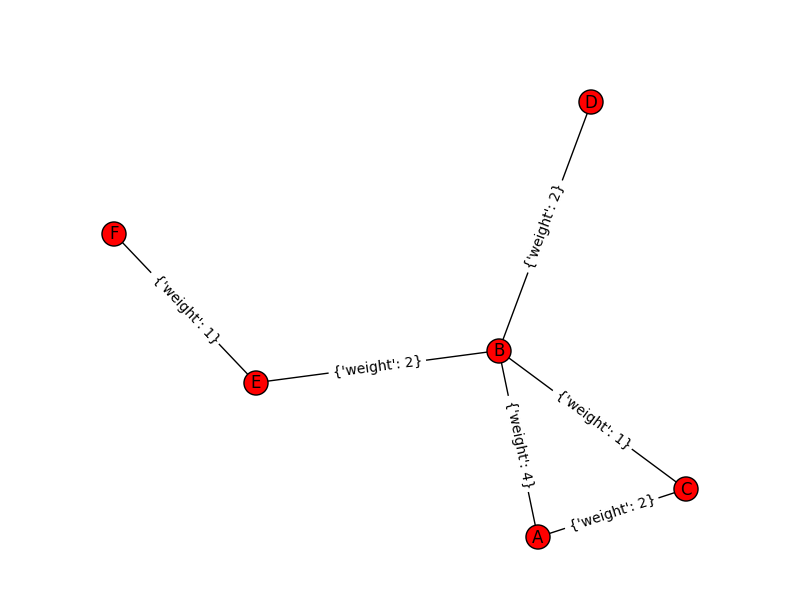# Laplacian Centrality in NetworkX (Python)

The other day I read a few papers on a new algorithm for calculating centrality in networks. Below are the two papers describing the Laplacian Centrality metric. The first is for non-weighted networks, and the second for weighted networks.

• Qi, X., Duval, R. D., Christensen, K., Fuller, E., Spahiu, A., Wu, Q., Wu, Y., Tang, W., and Zhang, C. (2013). Terrorist networks, network energy and node removal: A new measure of centrality based on laplacian energy. Social Networking, 02(01):19-31.
• Qi, X., Fuller, E., Wu, Q., Wu, Y., and Zhang, C.-Q. (2012). Laplacian centrality: A new centrality measure for weighted networks. Information Sciences, 194:240-253. PDF Here.

The metric is fairly intuitive I think. The centrality parameter is a function of the local degree plus the degree’s of the neighbors (with different weights for each). I figured it would be a quick programming exercise (which means I spent way too long trying to implement it!). To follow is some code that replicates the measures for both weighted and non-weighted graphs, using the Python `networkx` library.

The non-weighted graph code is easy, and is a near copy-paste from some igraph code snippet that was already available. Just some updates to idiom’s for NetworkX specifically. The `norm` option specifies whether you want solely the numerator value, the difference between the energy in the full graph versus the graph with the node removed (`norm=False`), or whether you want to divide this value by the energy for the full graph. Note this function ignores the weights in the graph. `nbunch` is if you want to pass the function only a subset of points to calculate the centrality. (If you do that you might as well have `norm=False` for time savings as well.)

``````def lap_cent(graph, nbunch=None, norm=False):
if nbunch is None:
vs = graph.nodes()
else:
vs = nbunch
degrees = graph.degree(weight=None)
if norm is True:
den = sum(v**2 + v for i,v in degrees.items())
den = float(den)
else:
den = 1
result = []
for v in vs:
neis = graph.neighbors(v)
loc = degrees[v]
nei = 2*sum(degrees[i] for i in neis)
val = (loc**2 + loc + nei)/den
result.append(val)
return result``````

The weighted network is a bit more tricky though. I thought coding all of the two walks seemed a royal pain, so I developed a different algorithm that I believe is quicker. Here are three functions, but the last one is the one of interest, `lap_cent_weighted`. The options are similar to the unweighted version, with the exception that you can pass a weight attribute (which is by default named ‘weight’ in NetworkX graphs).

``````def lap_energy(graph, weight='weight'):
degrees = graph.degree(weight=weight)
d1 = sum(v**2 for i,v in degrees.items())
wl = 0
for i in graph.edges(data=True):
wl += (i.get(weight))**2
return [d1,2*wl]

def cw(graph,node,weight='weight'):
neis = graph.neighbors(node)
ne = graph[node]
cw,sub = 0,0
for i in neis:
we = ne[i].get(weight)
od = graph.degree(i,weight=weight)
sub += -od**2 + (od - we)**2
cw += we**2
return [cw,sub]

def lap_cent_weighted(graph, nbunch=None, norm=False, weight='weight'):
if nbunch is None:
vs = graph.nodes()
else:
vs = nbunch
if norm == True:
fe = lap_energy(graph,weight=weight)
den = float(fe+fe)
else:
den = 1
result = []
for i in vs:
d2 = graph.degree(i,weight=weight)
w2 = cw(graph,i,weight=weight)
fin = d2**2 - w2 + 2*w2
result.append(fin/den)
return result``````

For a brief overview of the new algorithm (in some quick and dirty text math), to define the energy of the entire graph it is:

``sum(di^2) + 2*sum(wij^2)   (1)``

Where di are the degrees for all i nodes, and the second term is 2 times the sum of the weights squared. So when you take out a particular node, say ‘A’, the drop in the second term is easy, just iterate over the neighbors of ‘A’, and calculate `2*sum(waj^2)`, then subtract that from the second term in equation 1.

The first term is slightly more complex. First there is a decrease due to simply the degree of the removed node, `da^2`. There is also a decrease in the degree on the neighboring nodes as well, so you need to calculate their updated contribution. The necessary info. is available when you iterate over the neighbor list though, and if the original contribution is `di^2`, and the weight of `wia`, then the updated weight is `-di^2 + (di - wia)^2`. You can calculate this term at the same time you calculate the decrease in the weights in the second term.

I believe this algorithm is faster than the one originally written in the second paper. It should be something like O(n*a), where a is the average number of neighbors for the entire graph, and n are the number of nodes. Or in worst case a is the maximum number of neighbors any node has in the graph (which should be less than or equal to the max degree in a weighted graph).

Here is an example of using the functions with the small, toy example in the weighted network paper. Note that the `lap_cent` function ignores the weights.

``````import networkx as nx

Gp = nx.Graph()
ed = [('A','B',4),('A','C',2),('C','B',1),('B','D',2),('B','E',2),('E','F',1)]

x = lap_cent(Gp)
xw = lap_cent_weighted(Gp, norm=True)
for a,b,c in zip(Gp.nodes(),x,xw):
print a,b,c``````

Which prints out at the console:

``````A 18 0.7
C 18 0.28
B 34 0.9
E 16 0.26
D 10 0.22
F 6 0.04``````

If you want to see that the graph is the same as the graph in the weighted Qi paper, use below.

``````import matplotlib.pyplot as plt
pos=nx.spring_layout(Gp) # positions for all nodes
nx.draw(Gp,pos=pos)
nx.draw_networkx_labels(Gp,pos=pos)
nx.draw_networkx_edge_labels(Gp,pos=pos)
plt.show()``````1.#### mohammed

/  October 16, 2017

Hello i need code to measure perfomance networkx

•